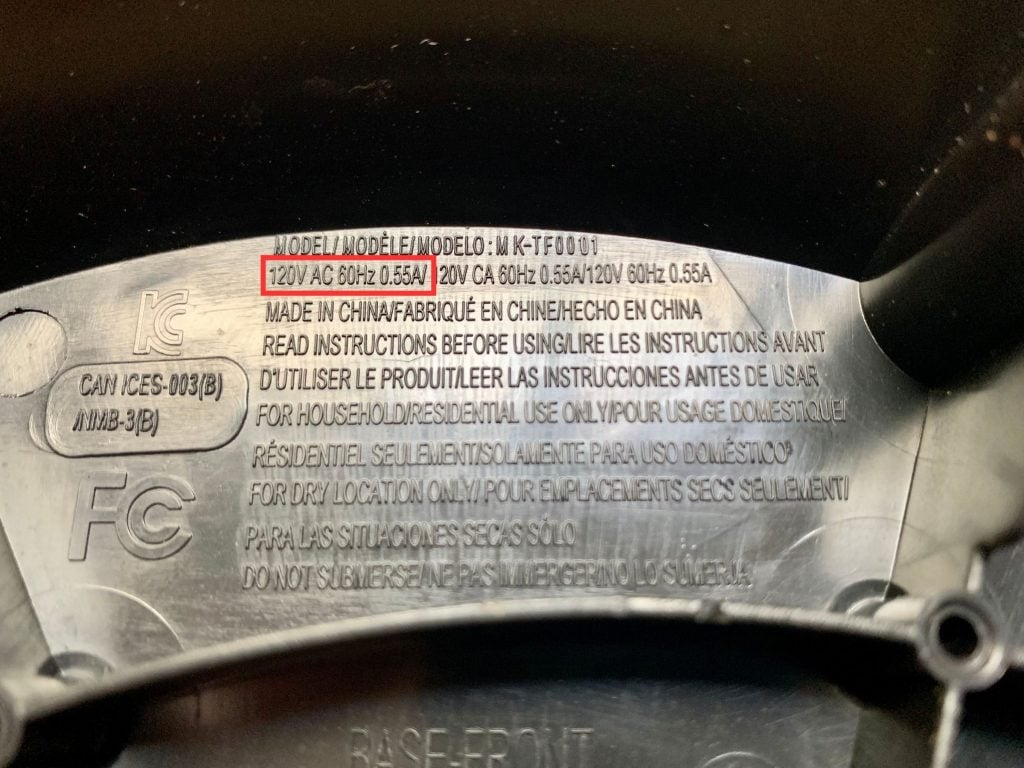# Off-Grid Solar Load (kWh) Calculator

Use our off-grid solar load calculator to easily calculate the total energy consumption in kilowatt hours (kWh) of the loads and appliances you want to power with solar.

Error: This field is required.
Error: This field is required and must be equal to or greater than 1.
Error: This field is required.
Error: This field is required.

Total energy consumption:
kWh per day

## How to Calculate Total Energy Consumption for an Off-Grid Solar System

The first step in sizing an off-grid solar system is figuring out how much energy all your devices and appliances (aka "loads") use. This process is sometimes called an "energy audit".

Our solar load calculator at the top of this page helps you do this, but here's the process broken down step by step:

1. List out everything you want to power with your solar system. Let’s say you’re planning to solar power a small off-grid cabin. Your list might look something like this:

• Mini fridge
• TV
• 6 LED light bulbs
• Microwave
• Electric cooktop
• Water pump
• 2 fans

2. Find the average power consumption in watts for each load. The easiest way to do this is to find the listed wattage on the product's electrical specifications label. If the device only lists its amps, you can multiply amps by volts to get watts.

For instance, here's the electrical specs label on a tower fan I own:My fan's electrical specs don't tell me how many watts it uses, but they do tell me that it uses 0.55A at 120V. So I can multiply 0.55A × 120V to get 66W.

3. List out the average amount of time each device or appliance is on per day. So, after finding each load's wattage and time on per day, your list might look something like this:

4. Find the daily energy consumption in watt hours (Wh) of each load by multiplying the power consumption by the time on per day and then by the quantity. If you know the time on per day in minutes, convert it to hours by dividing by 60.

For example, here's how you'd calculate the power consumption for the LED light bulbs listed in the above table:

```Load energy consumption = Avg Power Consumption (W) × Time On per Day (hrs) × Quantity.

Load energy consumption = 10 W × 4 hrs × 4

Load energy consumption = 160 Wh per day```

Tip: For loads that consume variable amounts of power, such as fridges, I recommend using an electricity usage monitor -- like a Kill-a-Watt or a Kasa Smart Plug with Energy Monitoring -- to get a much more accurate estimate of their daily energy usage.

5. Once you've found the daily energy consumption of all your loads, add them all together to get your total daily energy consumption. After doing the calculations for my list of loads, I got a total energy consumption of 1,571 Wh per day.

6. If desired, convert the total daily energy consumption from watt hours to kilowatt hours (kWh) by dividing by 1,000. Kilowatt hours are a more common unit for expressing energy usage in larger off-grid solar systems.

```Total energy consumption (kWh) = Total energy consumption (Wh) ÷ 1,000

Total energy consumption (kWh) = 1,571 Wh per day ÷ 1,000

Total energy consumption (kWh) = 1.571 kWh per day```

7. Write this number down and save it for later. It's a critical number for sizing your solar system.

And with that, you have an estimate of how much energy your off-grid solar power system will consume each day.

Congrats! You're done with the first key step of designing your system.

The next step is to size your battery bank, if you don't already have one.

To do that, continue to my off-grid solar battery calculator.